Win up to 100% scholarship on Aakash BYJU'S JEE/NEET courses with ABNAT Win up to 100% scholarship on Aakash BYJU'S JEE/NEET courses with ABNAT

# JEE Main 2022 July 26 – Shift 2 Physics Question Paper with Solutions

The JEE Main 2022 July 26 – Shift 2 Physics Question Paper with Solutions is given on this page. Students can use the JEE Main 2022 answer keys to scrutinise their performance in the JEE Main 2022 exam. They can use the JEE Main 2022 question paper with solutions to be familiar with the exam pattern and type of questions asked. The JEE Main 2022 question papers’ solutions will help them to find the complete solution in a step-by-step method.

## JEE Main 2022 July 26th Shift 2 Physics Question Paper and Solutions

SECTION – A

Multiple Choice Questions: This section contains 20 multiple choice questions. Each question has 4 choices (1), (2), (3) and (4), out of which ONLY ONE is correct.

1. Two projectiles are thrown with same initial velocity making an angle of 45° and 30° with the horizontal, respectively. The ratio of their respective ranges will be

$$\begin{array}{l} (\text{A})\ 1:\sqrt{2} \end{array}$$
$$\begin{array}{l} (\text{B})\ \sqrt{2}:1 \end{array}$$
$$\begin{array}{l} (\text{C})\ 2:\sqrt{3}\end{array}$$
$$\begin{array}{l} (\text{D})\ \sqrt{3}:2\end{array}$$

Sol.

$$\begin{array}{l} R=\frac{u^2\sin2\theta}{g}\end{array}$$
$$\begin{array}{l} \Rightarrow\ \frac{R_1}{R_2}=\frac{\sin2\theta_1}{\sin2\theta_2}=\frac{1}{\frac{\sqrt{3}}{2}}=\frac{2}{\sqrt{3}} \end{array}$$

2. In a Vernier Calipers, 10 divisions of Vernier scale is equal to the 9 divisions of main scale. When both jaws of Vernier calipers touch each other, the zero of the Vernier scale is shifted to the left of zero of the main scale and 4th Vernier scale division exactly coincides with the main scale reading. One main scale division is equal to 1 mm. While measuring diameter of a spherical body, the body is held between two jaws. It is now observed that zero of the Vernier scale lies between 30 and 31 divisions of main scale reading and 6th Vernier scale division exactly coincides with the main scale reading. The diameter of the spherical body will be

(A) 3.02 cm

(B) 3.06 cm

(C) 3.10 cm

(D) 3.20 cm

Sol. 10 VSD = 9 MSD

$$\begin{array}{l} \Rightarrow\ \text{LC}=\frac{1}{10}\text{MSD}=0.01\text{ cm}\end{array}$$

Negative error = (0.1 – 0.04) cm = 0.06 cm

Reading = (3.0 cm) + 6(0.01) cm + 0.06 cm

= 3.12 cm

Closer to 3.10 cm

3. A ball of mass 0.15 kg hits the wall with its initial speed of 12 ms–1 and bounces back without changing its initial speed. If the force applied by the wall on the ball during the contact is 100 N, calculate the time duration of the contact of ball with the wall.

(A) 0.018 s

(B) 0.036 s

(C) 0.009 s

(D) 0.072 s

Sol. F = 100 N

ΔP = 2 × 0.15 × 12

= 3.6

$$\begin{array}{l} \Rightarrow\ t=\frac{3.6}{100}=0.036\text{ s} \end{array}$$

4. A body of mass 8 kg and another of mass 2 kg are moving with equal kinetic energy. The ratio of their respective momenta will be

(A) 1:1

(B) 2:1

(C) 1:4

(D) 4:1

Sol.

$$\begin{array}{l} P=\sqrt{2m\ KE}\end{array}$$
$$\begin{array}{l} \Rightarrow\ \frac{P_1}{P_2}=\sqrt{\frac{m_1}{m_2} }\end{array}$$
$$\begin{array}{l} =\sqrt{\frac{8}{2}}=\frac{2}{1} \end{array}$$

5. Two uniformly charged spherical conductors, A and B of radii 5 mm and 10 mm are separated by a distance of 2 cm. If the spheres are connected by a conducting wire, then in equilibrium condition, the ratio of the magnitude of the electric fields at surface of the spheres A and B will be

(A) 1:2

(B) 2:1

(C) 1:1

(D) 1:4

Sol. After connection

$$\begin{array}{l}\sigma _1R_1 = \sigma _2R_2\end{array}$$

Now

$$\begin{array}{l} E=\frac{\sigma}{\varepsilon_0}\end{array}$$
$$\begin{array}{l} \Rightarrow\ \frac{E_1}{E_2}=\frac{\sigma_1}{\sigma_2}=\frac{R_2}{R_1} \end{array}$$
$$\begin{array}{l} =\frac{2}{1}\end{array}$$

6. The oscillating magnetic field in a plane electromagnetic wave is given by By = 5 × 10–6 sin1000π(5x – 4 × 108t)T. The amplitude of electric field will be:

(A) 15 × 102Vm–1

(B) 5 × 10–6Vm–1

(C) 16 × 1012Vm–1

(D) 4 × 102Vm–1

Sol. Speed of light

$$\begin{array}{l} c=\frac{\omega}{k}=\frac{4\times10^8}{5}=0.8\times10^8\text{ m/sec} \end{array}$$

so E0 = cB0

= 0.8 × 108 × 5 × 10–6

= 400 V/m

= 4 × 10–2

7. Light travels in two media M1 and M2 with speeds 1.5 × 108ms–1 and 2.0 × 108ms–1, respectively. The critical angle between them is:

$$\begin{array}{l} (\text{A})\ \tan^{-1}\left(\frac{3}{\sqrt{7}}\right)\end{array}$$
$$\begin{array}{l} (\text{B})\ \tan^{-1}\left(\frac{2}{3}\right)\end{array}$$
$$\begin{array}{l} (\text{C})\ \cos^{-1}\left(\frac{3}{4}\right)\end{array}$$
$$\begin{array}{l} (\text{D})\ \sin^{-1}\left(\frac{2}{3}\right)\end{array}$$

Sol. Critical angle between them

$$\begin{array}{l} \sin i_c=\frac{\mu_2}{\mu_1}=\frac{v_1}{v_2}\end{array}$$
$$\begin{array}{l} \sin i_c=\frac{3}{4}\end{array}$$
$$\begin{array}{l} \Rightarrow\ \tan i_c=\frac{3}{\sqrt{7}}\end{array}$$
$$\begin{array}{l} i_c=\tan^{-1}\frac{3}{\sqrt{7}}\end{array}$$

8. A body is projected vertically upwards from the surface of earth with a velocity equal to one third of escape velocity. The maximum height attained by the body will be:

(Take radius of earth = 6400 km and g = 10 ms–2)

(A) 800 km

(B) 1600 km

(C) 2133 km

(D) 4800 km

Sol. Applying conservation of energy

$$\begin{array}{l} -\frac{GM_em}{R_e}+\frac{1}{2}m\left(\frac{1}{3}\sqrt{\frac{2Gm_e}{R_e}}\right)^2=-\frac{GM_em}{R_e+h} \end{array}$$
$$\begin{array}{l} -\frac{GM_em}{R_e}+\frac{GM_em}{9R_e}=-\frac{GM_em}{R_e+h}\end{array}$$
$$\begin{array}{l} \frac{8}{9R_e}=\frac{1}{R_e+h} \end{array}$$
$$\begin{array}{l} \Rightarrow\ h=\frac{R_e}{8}=\frac{6400}{8}=800\text{ km}\end{array}$$

9. The maximum and minimum voltage of an amplitude modulated signal are 60 V and 20 V, respectively. The percentage modulation index will be:

(A) 0.5%

(B) 50%

(C) 2%

(D) 30%

Sol. Percentage modulation

$$\begin{array}{l} \mu=\frac{V_\text{max}-V_{\text{min}}}{V_\text{max}+V_\text{min}}\times 100\end{array}$$
$$\begin{array}{l} =\frac{60-20}{60+20}\times100 \\= 50\%\end{array}$$

10. A nucleus of mass M at rest splits into two parts having masses

$$\begin{array}{l} \frac{M’}{3}\ \text{and}\ \frac{2M’}{3}\left(M'<M\right).\end{array}$$
. The ratio of de Broglie wavelength of two parts will be:

(A) 1 : 2

(B) 2 : 1

(C) 1 : 1

(D) 2 : 3

Sol. Linear momentum is conserved

$$\begin{array}{l}\text{so}~ p_{M’/3}=p_{2M’/3}\end{array}$$
$$\begin{array}{l}\text{so}~ \frac{\lambda_{M’/3}}{\lambda_{2M’/3}}=\frac{1}{1}\end{array}$$

11. An ice cube of dimensions 60 cm × 50 cm × 20 cm is placed in an insulation box of wall thickness 1 cm. The box keeping the ice cube at 0°C of temperature is brought to a room of temperature 40°C. The rate of melting of ice is approximately.

(Latent heat of fusion of ice is 3.4 × 105 J kg–1 and thermal conducting of insulation wall is 0.05 Wm–1°C–1)

$$\begin{array}{l}\left(A\right) 61 \times 10^{-3} kg s^{-1}\end{array}$$
$$\begin{array}{l}\left(A\right) 61 \times 10^{-5} kg s^{-1}\end{array}$$
$$\begin{array}{l}\left(A\right) 208 kg s^{-1}\end{array}$$
$$\begin{array}{l}\left(A\right) 30 \times 10^{-5} kg s^{-1}\end{array}$$

Sol.

$$\begin{array}{l} \frac{\Delta Q}{\Delta t}=\frac{kA\left(T_1-T_2\right)}{\ell}\end{array}$$
$$\begin{array}{l} \Rightarrow\ \frac{mL}{\Delta t}=\frac{kA\left(T_1-T_2\right)}{\ell}\end{array}$$
$$\begin{array}{l} \Rightarrow\ \frac{m}{\Delta t}=\frac{kA\left(T_1-T_2\right)}{L\ell}\end{array}$$
$$\begin{array}{l} \simeq\ 61.1\times10^{-5}\text{ kg/s}\end{array}$$

12. A gas has n degrees of freedom. The ratio of specific heat of gas at constant volume to the specific heat of gas at constant pressure will be

$$\begin{array}{l} (\text{A})\ \frac{n}{n+2} \end{array}$$
$$\begin{array}{l} (\text{B})\ \frac{n+2}{n} \end{array}$$
$$\begin{array}{l} (\text{C})\ \frac{n}{2n+2} \end{array}$$
$$\begin{array}{l} (\text{D})\ \frac{n}{n-2} \end{array}$$

Sol.

$$\begin{array}{l} C_V=\frac{nR}{2} \end{array}$$

And

$$\begin{array}{l} C_P=\frac{nR}{2}+R\end{array}$$
$$\begin{array}{l} \Rightarrow\ \frac{C_V}{C_P}=\frac{\frac{nR}{2}}{\frac{nR}{2}+R}=\frac{n}{n+2}\end{array}$$

13. A transverse wave is represented by y = 2sin(ωtkx) cm. The value of wavelength (in cm) for which the wave velocity becomes equal to the maximum particle velocity, will be

(A) 4π

(B) 2π

(C) π

(D) 2

Sol.

$$\begin{array}{l} \frac{\omega}{k}=A\omega\end{array}$$
$$\begin{array}{l} \Rightarrow\ k=\frac{1}{A}=\frac{1}{2\text{ cm}} \end{array}$$
$$\begin{array}{l} \Rightarrow\ \frac{2\pi}{\lambda}=\frac{1}{2\text{ cm}}\end{array}$$
$$\begin{array}{l}\Rightarrow \lambda = 4\pi\text{cm} \end{array}$$

14. A battery of 6 V is connected to the circuit as shown below. The current I drawn from the battery is$$\begin{array}{l}\left(A\right) 1 A\end{array}$$
$$\begin{array}{l}\left (B\right ) 2 A\end{array}$$
$$\begin{array}{l} \left(C\right) \frac{6}{11}\text{ A}\end{array}$$
$$\begin{array}{l} \left(D\right) \frac{4}{3}\text{ A}\end{array}$$

Sol.

$$\begin{array}{l}\text{Balanced Wheatstone}\Rightarrow\ R_\text{eff}=\frac{3\times6}{3+6}\times2+2\\= 6 ~\Omega\end{array}$$
$$\begin{array}{l} \Rightarrow\ I=\frac{V}{R}=1\text{A}\end{array}$$

15. A source of potential difference V is connected to the combination of two identical capacitors as shown in the figure. When key ‘K’ is closed, the total energy stored across the combination is E1. Now key ‘K’ is opened and dielectric of dielectric constant 5 is introduced between the plates of the capacitors. The total energy stored across the combination is now E2. The ratio E1/E2 will be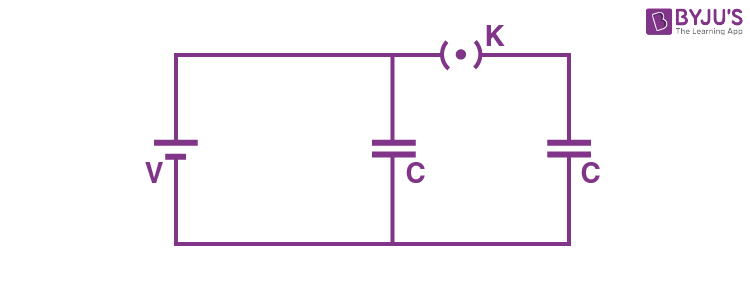$$\begin{array}{l} (\text{A})\ \frac{1}{10}\end{array}$$
$$\begin{array}{l} (\text{B})\ \frac{2}{5}\end{array}$$
$$\begin{array}{l} (\text{C})\ \frac{5}{13}\end{array}$$
$$\begin{array}{l} (\text{D})\ \frac{5}{26}\end{array}$$

Sol.

$$\begin{array}{l} E_1 = \frac{1}{2}\left(2C\right)V^2 \\ \Rightarrow E_1 = CV^2….(i)\end{array}$$
$$\begin{array}{l} E_2=\frac{1}{2}\left(5C\right)V^2+\frac{1}{2}\frac{\left(CV\right)^2}{5C} \end{array}$$
$$\begin{array}{l} =\frac{13}{5}CV^2\end{array}$$
$$\begin{array}{l} \Rightarrow\ \frac{E_1}{E_2}=\frac{5}{13}\end{array}$$

16. Two concentric circular loops of radii r1 = 30 cm and r2 = 50 cm are placed in XY plane as shown in the figure. A current I = 7 A is flowing through them in the direction as shown in figure. The net magnetic moment of this system of two circular loops is approximately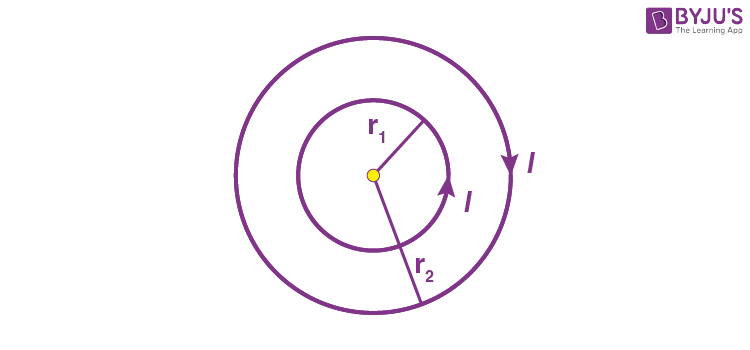$$\begin{array}{l} (\text{A})\ \frac{7}{2}\hat{k}\text{ Am}^2 \end{array}$$
$$\begin{array}{l} (\text{B})\ -\frac{7}{2}\hat{k}\text{ Am}^2 \end{array}$$
$$\begin{array}{l} (\text{C})\ 7\hat{k}\text{ Am}^2 \end{array}$$
$$\begin{array}{l} (\text{D})\ -7\hat{k}\text{ Am}^2 \end{array}$$

Sol.

$$\begin{array}{l} \mu_1=\pi r_1^2\times I_1\end{array}$$
$$\begin{array}{l} \mu_2=\pi r_2^2\times I_2\end{array}$$
$$\begin{array}{l} \therefore\ \mu_\text{net}=\left(\mu_2-\mu_1\right)\left(-\hat{k}\right) \end{array}$$
$$\begin{array}{l} =\pi\left(r_2^2-r_1^2\right)I\left(-\hat{k}\right)\end{array}$$
$$\begin{array}{l} =3.142\times\left(0.5^2-0.3^2\right)\times7\left(-\hat{k}\right)\end{array}$$
$$\begin{array}{l} =-\frac{7}{2}\hat{k}\text{ Am}^2 \end{array}$$

17. A velocity selector consists of electric field

$$\begin{array}{l} \vec{E}=E\hat{k}\ \text{and magnetic field}\ \vec{B}=B\hat{j}\end{array}$$
with B = 12 mT. The value of E required for an electron of energy 728 eV moving along the positive x-axis to pass undeflected is

(Given, mass of electron = 9.1 × 10–31 kg)

(A) 192 kVm–1

(B) 192 mVm–1

(C) 9600 kVm–1

(D) 16 kVm–1

Sol.

$$\begin{array}{l} v=\frac{E}{B}\end{array}$$
and
$$\begin{array}{l} K=\frac{1}{2}mv^2\end{array}$$
$$\begin{array}{l} \Rightarrow\ \sqrt{\frac{2K}{m}}\times B= E\end{array}$$
$$\begin{array}{l} \Rightarrow\ E=\sqrt{\frac{2\times728\times1.6\times10^{-19}}{9.1\times10^{-31}}}\times 12\times10^{-3}\\= 192000 ~\text{V/m}\end{array}$$

18. Two masses M1 and M2 are tied together at the two ends of a light inextensible string that passes over a frictionless pulley. When the mass M2 is twice that of M1, the acceleration of the system is a1. When the mass M2 is thrice that of M1, the acceleration of the system is a2. The ratio a1/a2 will be$$\begin{array}{l} (\text{A})\ \frac{1}{3}\end{array}$$
$$\begin{array}{l} (\text{B})\ \frac{2}{3}\end{array}$$
$$\begin{array}{l} (\text{C})\ \frac{3}{2}\end{array}$$
$$\begin{array}{l} (\text{D})\ \frac{1}{2}\end{array}$$

Sol.

$$\begin{array}{l} a_1=\frac{M_2-M_1}{M_2+M_1}\times g=\frac{2M_1-M_1}{3M_1}\times g \end{array}$$
$$\begin{array}{l} =\frac{g}{3}\end{array}$$

And,

$$\begin{array}{l} a_2=\frac{3M_1-M_1}{4M_1}\times g=\frac{g}{2} \end{array}$$
$$\begin{array}{l} \therefore\ \frac{a_1}{a_2}=\frac{g/3}{g/2}=\frac{2}{3} \end{array}$$

19. Mass numbers of two nuclei are in the ratio of 4 : 3. Their nuclear densities will be in the ratio of

(A) 4 : 3

$$\begin{array}{l} (\text{B})\ \left(\frac{3}{4}\right)^\frac{1}{3}\end{array}$$

(C) 1 : 1

$$\begin{array}{l} (\text{D})\ \left(\frac{4}{3}\right)^\frac{1}{3}\end{array}$$

Sol.

$$\begin{array}{l} \therefore\ R=R_0A^\frac{1}{3}\end{array}$$
$$\begin{array}{l} \Rightarrow\ \frac{R_1}{R_2}=\left(\frac{A_1}{A_2}\right)^\frac{1}{3}=\left(\frac{4}{3}\right)^\frac{1}{3} \end{array}$$
$$\begin{array}{l}\therefore \text{Density ratio,} \frac{\rho_1}{\rho_2}=\frac{A_1/V_1}{A_2/V_2}\end{array}$$
$$\begin{array}{l} =\left(\frac{A_1}{A_2}\right)\times\left(\frac{R_2}{R_1}\right)^3 \\= 1 \colon 1\end{array}$$

20. The area of cross section of the rope used to lift a load by a crane is 2.5 × 10–4 m2. The maximum lifting capacity of the crane is 10 metric tons. To increase the lifting capacity of the crane to 25 metric tons, The required area of cross section of the rope should be

(take g = 10 ms–2)

(A) 6.25 × 10–4 m2

(B) 10 × 10–4 m2

(C) 1 × 10–4 m2

(D) 1.67 × 10–4 m2

Sol.

$$\begin{array}{l} \frac{W_1}{A_1}=\frac{W_2}{A_2}\end{array}$$
$$\begin{array}{l} \Rightarrow\ A_2=\frac{W_2}{W_1}A_1\end{array}$$
$$\begin{array}{l} =\frac{25}{10}\times 2.5\times 10^{-4} \end{array}$$
$$\begin{array}{l}= 6.25 \times 10^{-4} \text{m}^2 \end{array}$$

SECTION – B

Numerical Value Type Questions: This section contains 10 questions. In Section B, attempt any five questions out of 10. The answer to each question is a NUMERICAL VALUE. For each question, enter the correct numerical value (in decimal notation, truncated/rounded-off to the second decimal place; e.g. 06.25, 07.00, –00.33, –00.30, 30.27, –27.30) using the mouse and the on-screen virtual numeric keypad in the place designated to enter the answer.

1. If

$$\begin{array}{l} \vec{A}=\left(2\hat{i}+3\hat{j}-\hat{k}\right)\text{m}\ \text{and}\ \vec{B}=\left(\hat{i}+2\hat{j}+2\hat{k}\right)\text{m}.\end{array}$$
The magnitude of component of vector
$$\begin{array}{l} \vec{A}\ \text{along vector}\ \vec{B}\end{array}$$
will be ______m.

Sol.

$$\begin{array}{l} A\cos\theta=\frac{\vec{A}.\vec{B}}{\left|\vec{B}\right|}=\frac{2+6-2}{3}=\frac{6}{3}=2\end{array}$$

2. The radius of gyration of a cylindrical rod about an axis of rotation perpendicular to its length and passing through the center will be_________m.

Given the length of the rod is 10√3 m.

Sol.

$$\begin{array}{l} I=\frac{ML^2}{12}=MK^2\end{array}$$
$$\begin{array}{l} K=\frac{L}{\sqrt{12}}=\frac{10\sqrt{3}}{\sqrt{12}}=5\text{ m}\end{array}$$

3. In the given figure, the face AC of the equilateral prism is immersed in a liquid of refractive index ‘n‘. For incident angle 60° at the side AC the refracted light beam just grazes along face AC. The refractive index of the liquid n = (√x)/4. The value of x is _______.

(Given refractive index of glass = 1.5)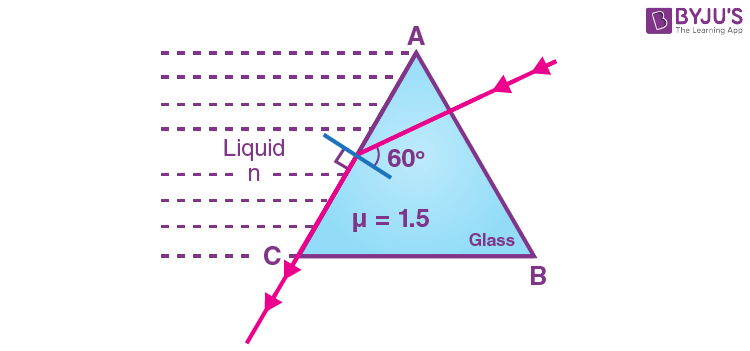Sol. 1.5 sin60° = 4 sin90°

$$\begin{array}{l} 1.5\times\frac{\sqrt{3}}{2}=\frac{3\sqrt{3}}{4}=\frac{\sqrt{27}}{4}=\frac{\sqrt{x}}{4} \end{array}$$

x = 27

4. Two lighter nuclei combine to from a comparatively heavier nucleus by the relation given below:

$$\begin{array}{l} _1^2X+_1^2X=_2^4Y\end{array}$$

The binding energies per nucleon for

$$\begin{array}{l} _1^2X\ \text{and}\ _2^4Y\end{array}$$
are 1.1 MeV and 7.6 MeV respectively. The energy released in the process is ______ MeV.

Sol. Energy released = Change in B.E.

(7.6 × 4) – [4 × 1.1] = 26 MeV

5. A uniform heavy rod of mass 20 kg, cross sectional area 0.4 m2 and length 20 m is hanging from a fixed support. Neglecting the lateral contraction, the elongation in the rod due to its own weight is x × 10–9 m. The value of x is _____

(Given Young’s modulus Y = 2 × 1011 Nm–2 and

g = 10 ms–2)

Sol.

$$\begin{array}{l} \frac{\frac{F}{A}}{\frac{\Delta L}{L}}=Y \end{array}$$
$$\begin{array}{l} \Delta L=\frac{FL}{AY}=\frac{T_\text{avg}L}{AY}=\frac{MgL}{2AY}\end{array}$$
$$\begin{array}{l} =\frac{20\times 10\times 20}{2\times 0.4\times2\times10^{11}}= \frac{4\times10^3\times10^{-11}}{4\times0.4}\end{array}$$
$$\begin{array}{l} = 2.5 \times 10^{-8} = 25 \times 10^{-9}\end{array}$$

6. The typical transfer characteristics of a transistor in CE configuration is shown in figure. A load resistor of 2 kΩ is connected in the collector branch of the circuit used. The input resistance of the transistor is 0.50 kΩ. The voltage gain of the transistor is ________.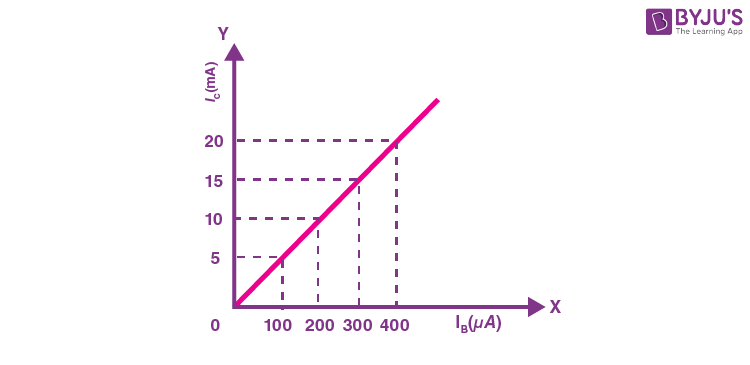Sol.

$$\begin{array}{l} V_\text{gain}=\text{Current gain}\times \frac{R_L}{R_i}\end{array}$$
$$\begin{array}{l} =\frac{\Delta I_C}{\Delta I_B}\times \frac{R_L}{R_i} \end{array}$$
$$\begin{array}{l} =\frac{5\times10^{-3}}{100\times10^{-6}}\times \frac{2\times10^3}{0.5\times10^3}=\frac{10}{0.5}\times 10=200\end{array}$$

7. Three point charges of magnitude 5 μC, 0.16μC and 0.3μC are located at the vertices, B, C of a right angled triangle whose sides are AB = 3 cm, BC = 3√2 cm and CA = 3 cm and point A is the right angle corner. Charge at point A, experiences ______N of electrostatic force due to the other two charges.

Sol.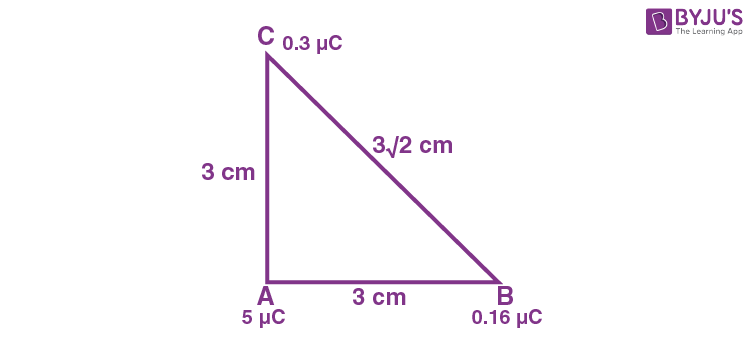$$\begin{array}{l} F_{AC}=\frac{k\left(5\times0.3\right)\times10^{-12}}{9\times10^{-4}} \end{array}$$
$$\begin{array}{l} F_{AB}=\frac{k\left(5\times0.16\right)\times10^{-12}}{9\times10^{-4}} \end{array}$$
$$\begin{array}{l} F_\text{net}=\frac{k\times10^{-12}}{9\times10^{-4}}\sqrt{1.5^2+\left(0.8\right)^2}\end{array}$$
$$\begin{array}{l} =\frac{10^9\times10^{-12}}{10^{-4}}\times 1.7=17 \end{array}$$

8. In a coil of resistance 8Ω, the magnetic flux due to an external magnetic field varies with time as

$$\begin{array}{l} \phi=\frac{2}{3}\left(9-t^2\right) \end{array}$$
. The value of total heat produced in the coil, till the flux becomes zero, will be ______ J.

Sol. R = 8Ω

$$\begin{array}{l}\phi=\frac{2}{3}\left(9-t^2\right)\end{array}$$

At t = 3, φ = 0

$$\begin{array}{l} \varepsilon=\left|-\frac{d\phi}{dt}\right|=\frac{4}{3}t \end{array}$$
$$\begin{array}{l} H=\int_0^3\frac{V^2}{R}dt=\int_0^3\frac{1}{8}\times\frac{16}{9}t^2dt\end{array}$$
$$\begin{array}{l} =\frac{2}{9}\times\left(\frac{t^3}{3}\right)_0^3=\frac{2}{9\times3}\times27=2\text{ J}\end{array}$$

9. A potentiometer wire of length 300 cm is connected in series with a resistance 780 Ω and a standard cell of emf 4V. A constant current flows through potentiometer wire. The length of the null point for cell of emf20 mV is found to be 60 cm. The resistance of the potentiometer wire is _____ Ω.

Sol.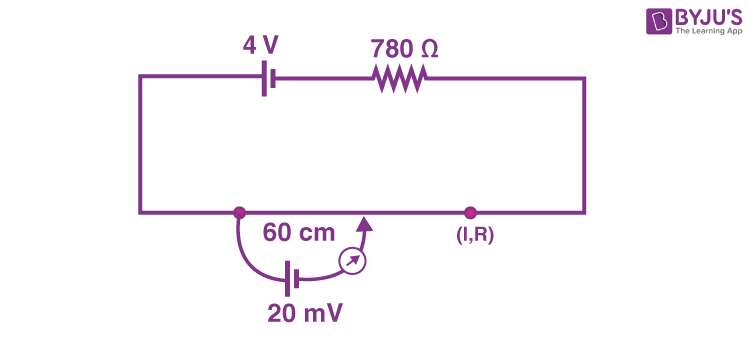l = 300 cm

ε = Kx

$$\begin{array}{l} 20\times10^{-3}=\left(\frac{4\times R}{780+R}\times\frac{1}{300}\right)60 \end{array}$$
$$\begin{array}{l} R=20 \end{array}$$

10. As per given figures, two springs of spring constants k and 2k are connected to mass m. If the period of oscillation in figure (a)is 3s, then the period of oscillation in figure (b) will be √x s. The value of x is _______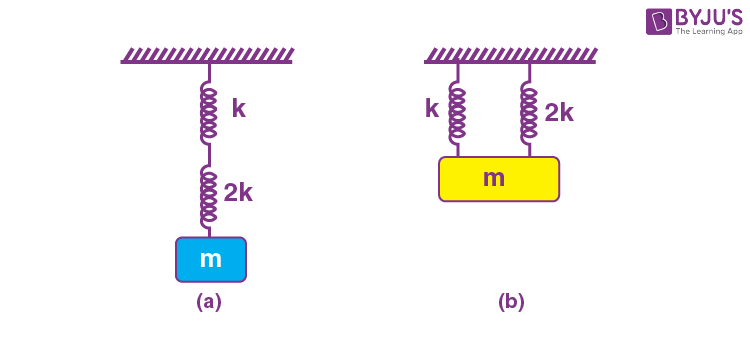Sol. For case (a),

$$\begin{array}{l} k_{eq}=\frac{2K}{3}\end{array}$$

For case (b),

$$\begin{array}{l} k_{eq}=3K\end{array}$$
$$\begin{array}{l} \because T=2\pi\sqrt{\frac{m}{k}}\end{array}$$
$$\begin{array}{l} \therefore\ \frac{T_a}{T_b}=\sqrt{\frac{K_b}{K_a}} \end{array}$$
$$\begin{array}{l} \frac{3}{T_b}=\sqrt{\frac{3K\times3}{2k}}=\frac{3}{\sqrt{2}} \end{array}$$
$$\begin{array}{l} T_b=\sqrt{2}\\x = 2 \end{array}$$

### Download PDF of JEE Main 2022 July 26 Shift 2 Physics Paper & Solutions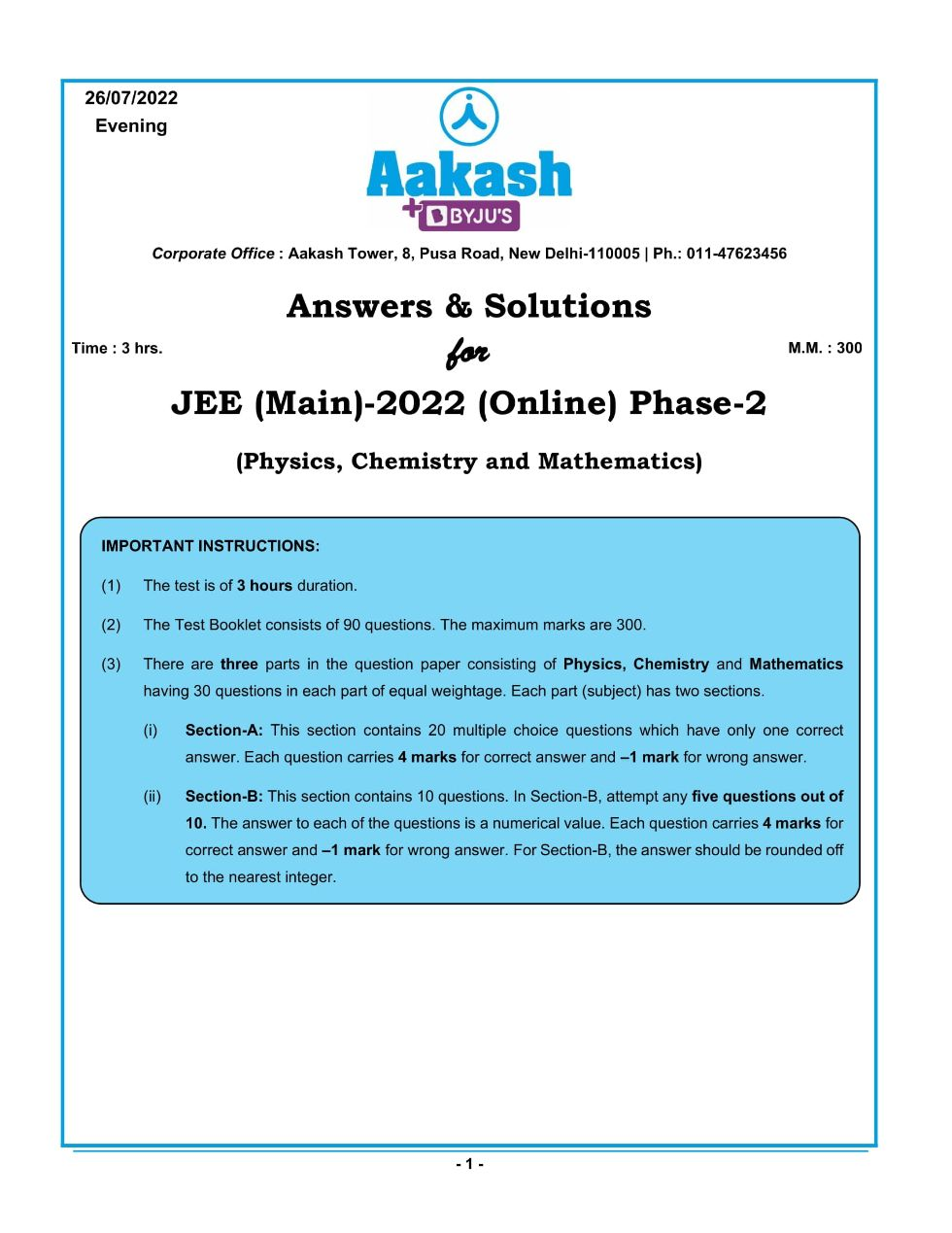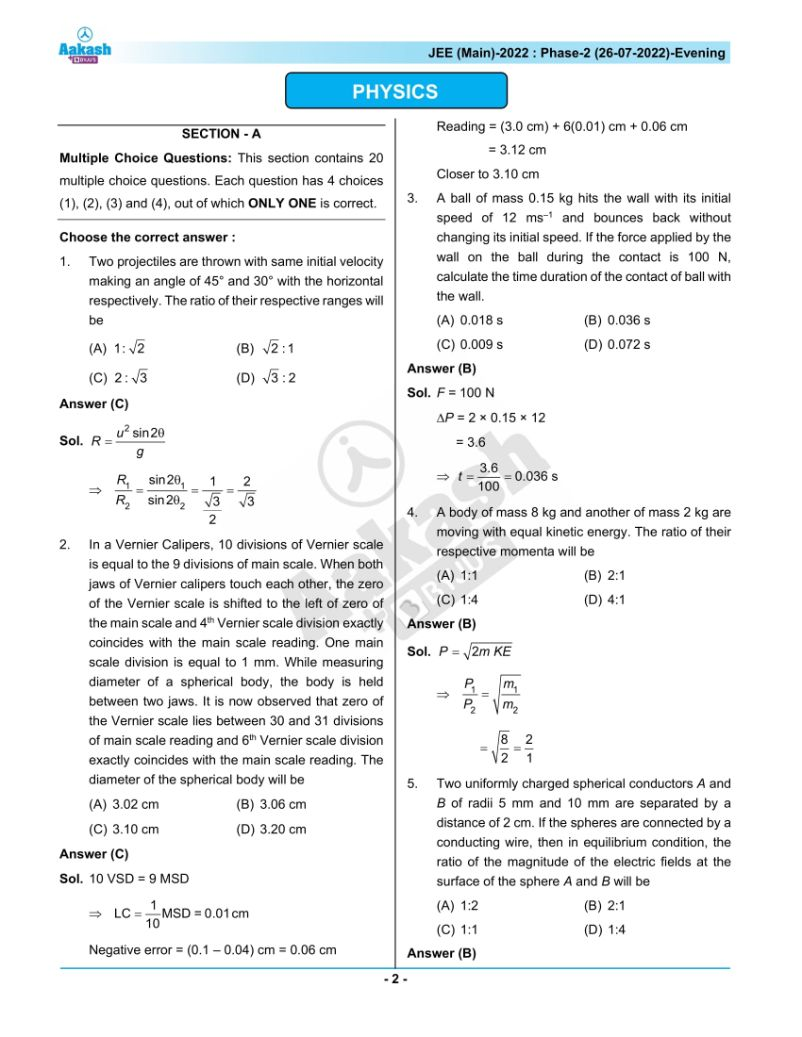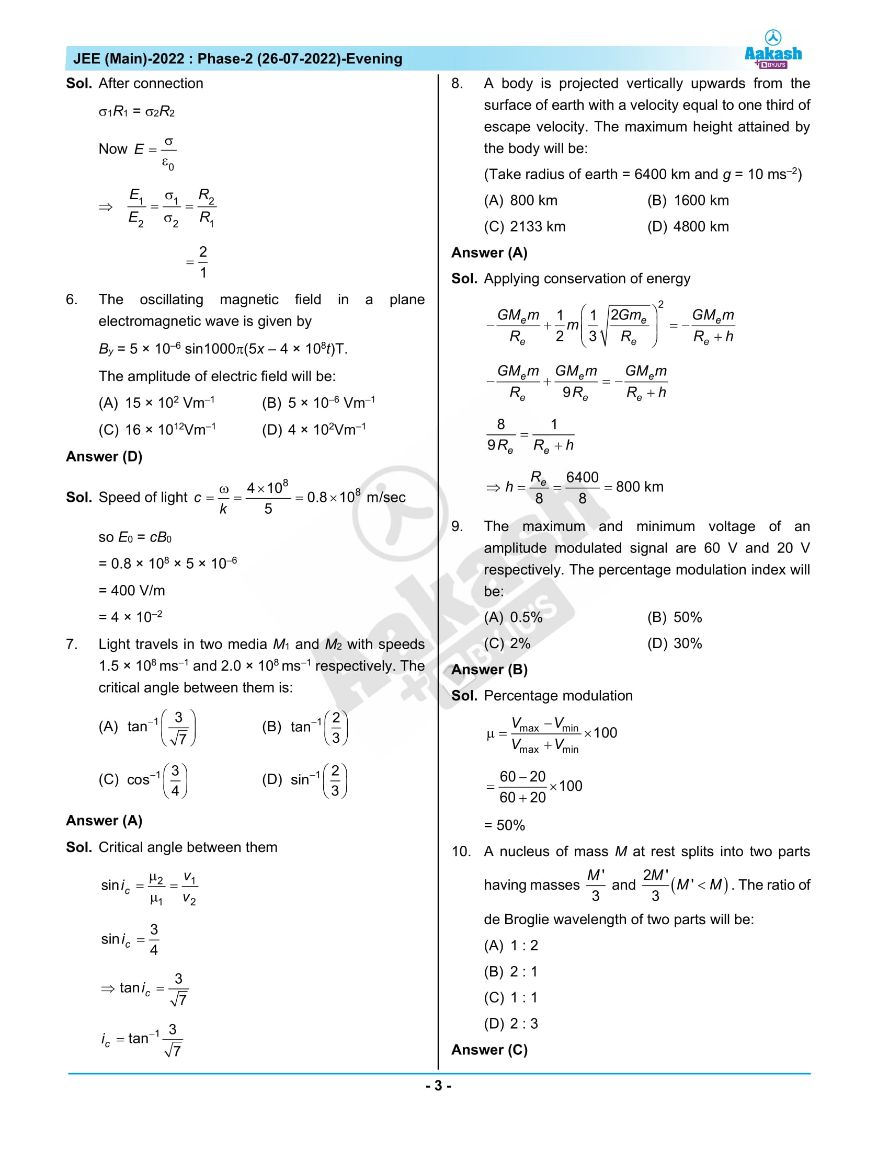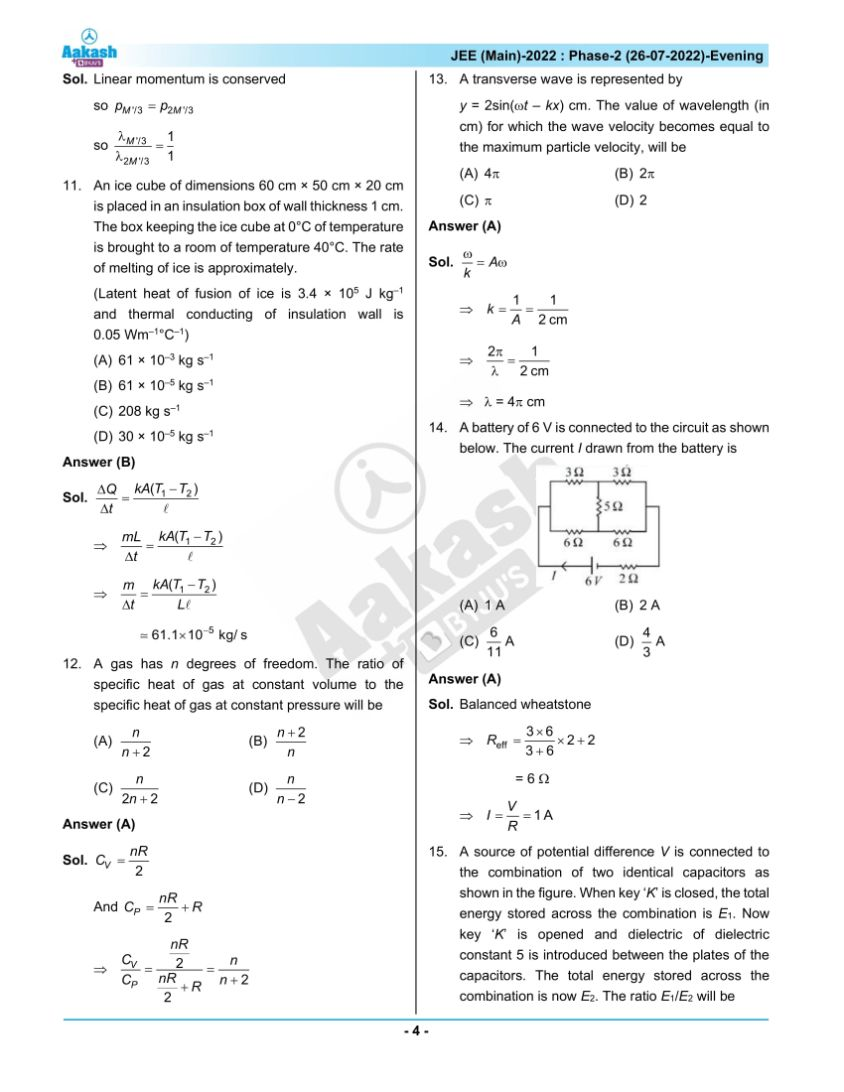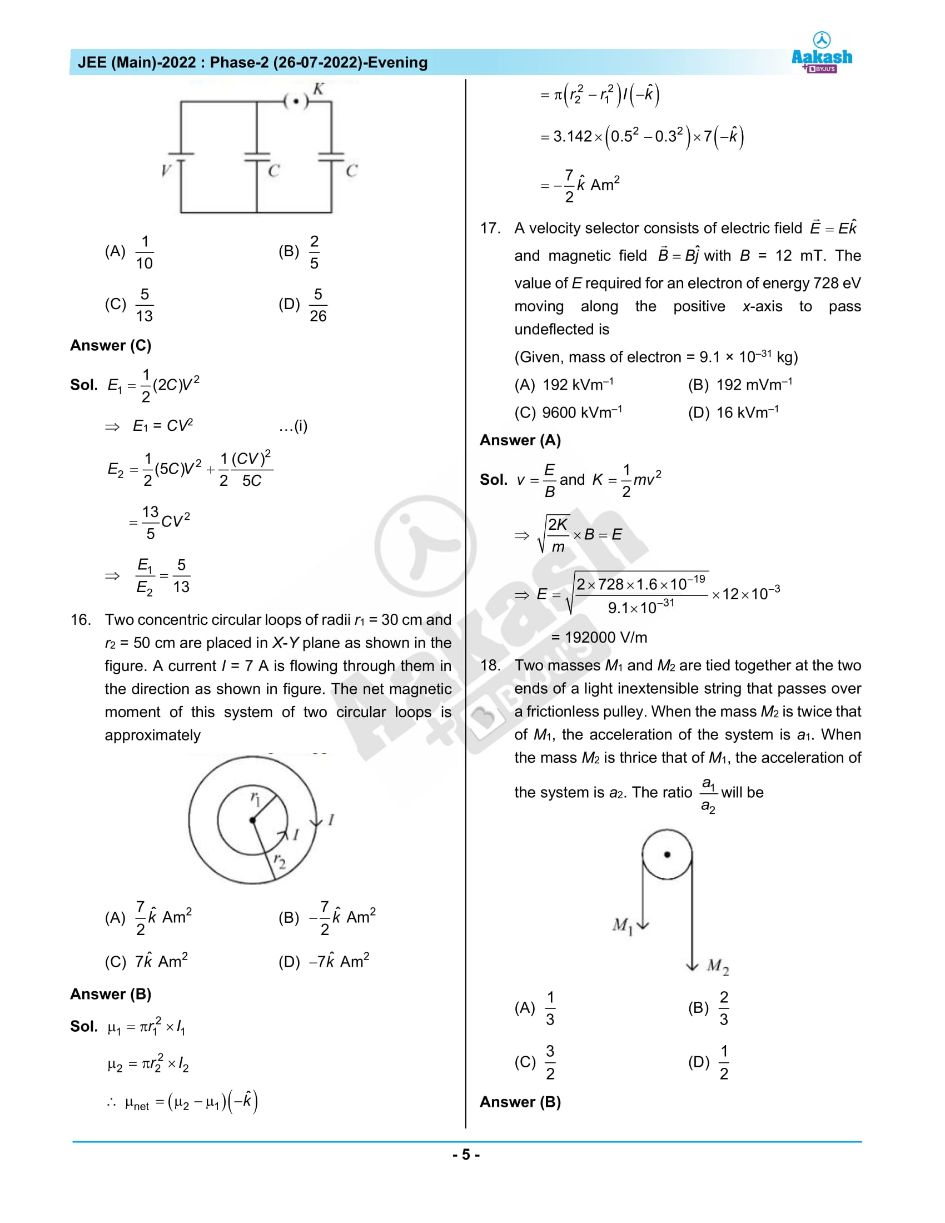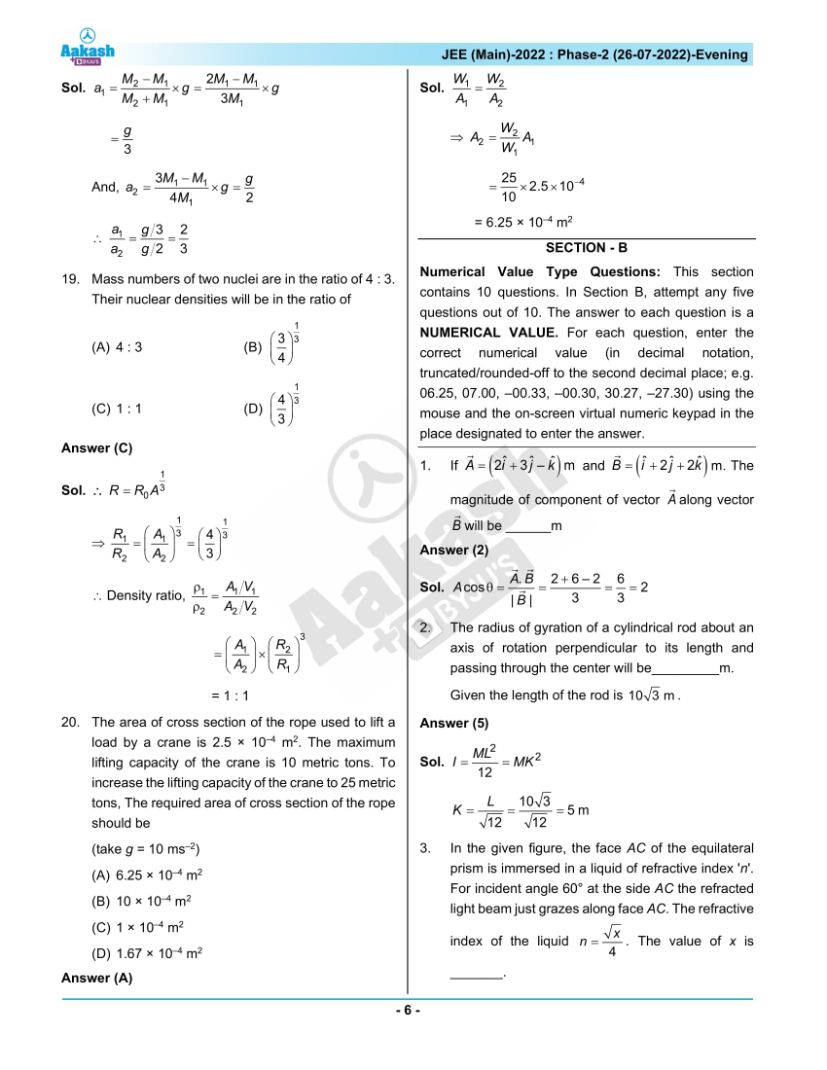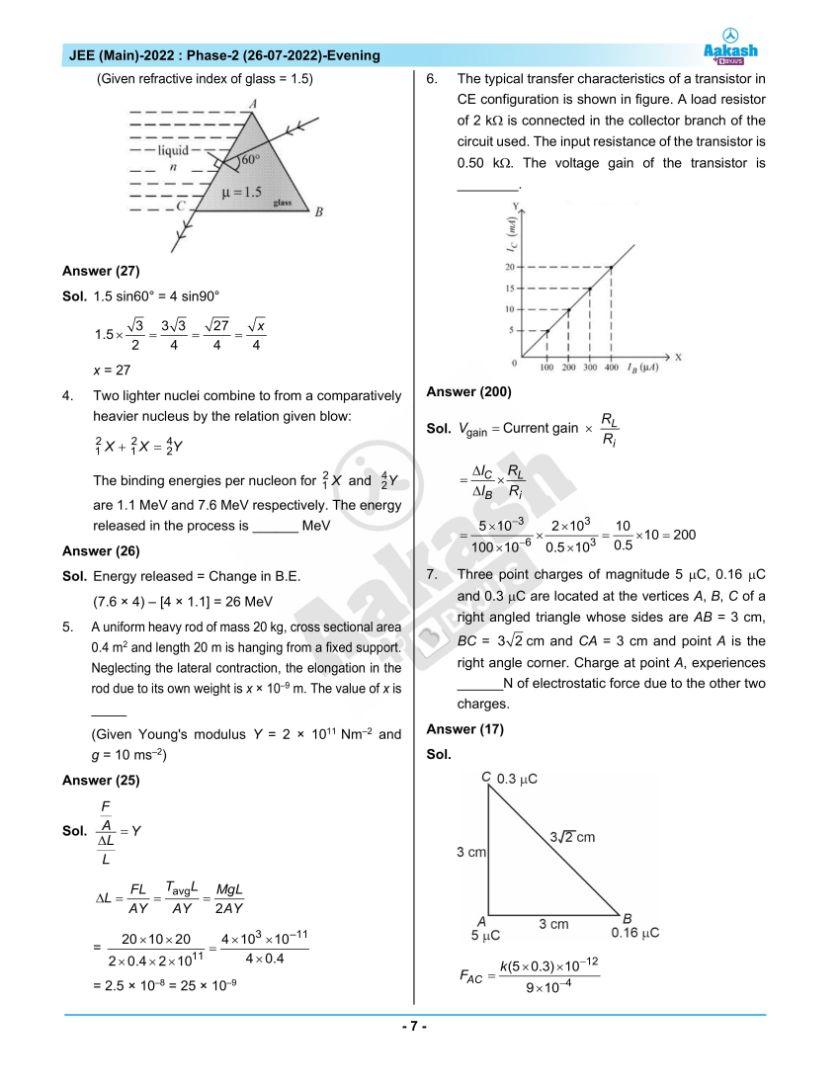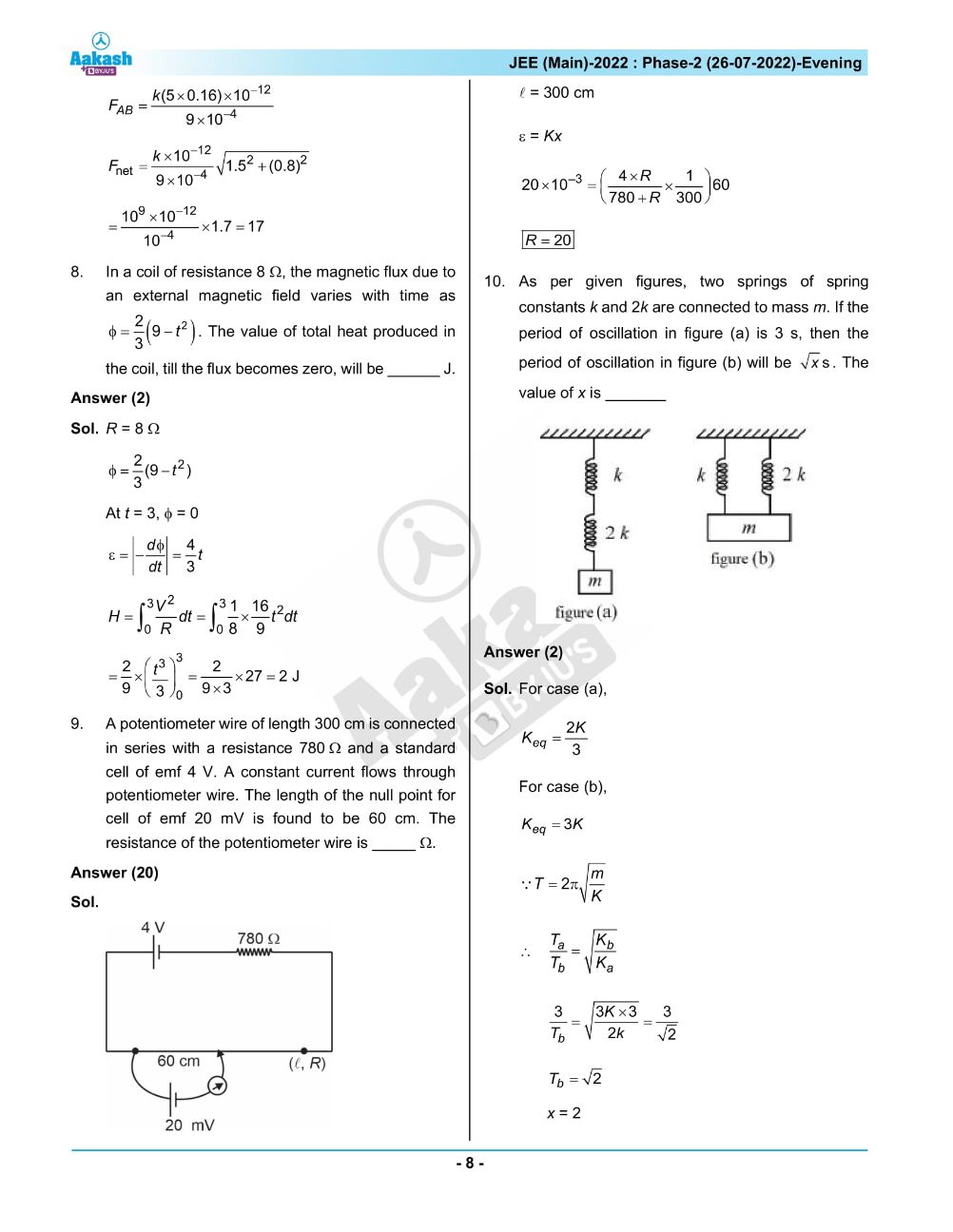## JEE Main 2022 July 26th Shift 2 Paper Analysis